Name:    Atoms and Periodic Table practice Quiz

Matching

Match each item with the correct statement below.
 a. proton d. electron b. nucleus e. neutron c. atom

1.

a subatomic particle with no charge that is found in the nucleus

2.

the smallest particle of an element that is comprised of smaller particles.

3.

the central part of an atom, containing protons and neutrons

4.

a negatively charged subatomic particle found outside the nucleus

5.

a positively charged subatomic particle that is found in the nucleus

Match each item with the correct statement below.
 a. mass number c. atomic number b. isotopes d. atomic mass

6.

atoms with the same number of protons, but different numbers of neutrons in the nucleus of an atom

7.

the number of protons in the nucleus of an element

8.

atoms of the same element with different numbers of neutrons

9.

the total number of protons and neutrons in the nucleus of an atom

Match each item with the correct statement below.
 a. group c. Mendeleev b. period d. Moseley

10.

horizontal row in the periodic table

11.

vertical column in the periodic table

12.

created the first periodic table and predicted undiscovered elements

13.

reordered the periodic table in order of increasing atomic number

Multiple Choice
Identify the choice that best completes the statement or answers the question.

14.

An atom has 12 protons and 13 neutrons. Which is the correct chemical symbol for this atom?
 a.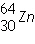b.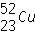c.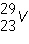d.15.

What is the mass number of the atom shown above?
 a. 19 b. 18 c. 11 d. 20

16.

Which is the correct symbol for this atom shown above?
 a.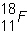1+ b.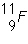c.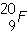1- d.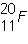1-

17.

The above figure is of a/an:
 a. anion b. isotope c. cation d. subion

18.

Which element has 14 protons?
 a. Si b. Al c. N d. S

19.

Which is the number of neutrons in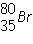?
 a. 80 b. 45 c. 115 d. 35

20.

Elements within a veritcal GROUP on the periodic table have similar properties because:
 a. they have the same number of valence electrons. b. they have the same the same number of energy levels. c. they have the same number of protons. d. they have the same number of neutrons.

21.

Most NONMETALS are
 a. ductile. b. good conductors of heat and electricity. c. gases at room temperature d. malleable.

22.

Which group of elements shares characteristics with both metals and nonmetals and are used to make semiconductors?
 a. salts b. metalloids c. halogens d. alloys

23.

Elements that are in the same PERIOD
 a. have the same number of valence electrons. b. have different numbers of energy levels. c. have the same number of energy levels. d. have similar properties.

Numeric Response
Place the following in the numerical order of discovery (1 being the earliest- 5 being the most recent):

24.

_____ Bohr- determined that electrons reside in energy levels that surround the nucleus

25.

_____ Rutherford: Discovered the positive nucleus and that atoms were mostly empty space.

26.

_____ Quantum mechanical model- Electrons reside within orbits in energy levels whose location is based on probability

27.

_____ Thomson- discovered the electron. Placed electrons randomly in a positive surrounding (blueberry muffin model)

28.

_____ Dalton- Atoms were solid speres with no smaller particles (like marbles)

Use the following for Questions 29-35

When Answering, MAKE SURE THAT YOU MATCH THE LETTER CASE EXACTLY29.

Which of the Symbols SHOWN is that of an Alkali Metal?

30.

Which of the Symbols SHOWN is that of a Noble Gas?

31.

Which of the Symbols SHOWN is that of a Halogen?

32.

Which of the Symbols SHOWN is that of an Alkaline Earth Metal?

33.

Which of the Symbols SHOWN is that of a Metalloidl?

34.

Which of the following will GAIN electrons to be like the nearest noble gas, Na or F?

35.

Which of the following will form a CATION, Na or F?